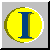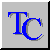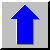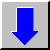Index Table of Contents previousChapter nextChapter

This option allows you to enter a constant value which is then added to the currently displayed map's grid data values. When a negative value is entered, that value is subtracted from each of the grid data values.

Expressed in mathematical terms:

result = current grid value + user-defined constant value.

The OK button closes this window after performing the calculations, and displays the new data values on the map in the current window.

The CANCEL button closes this window without performing any calculations or changing the displayed map's grid data values.

The HELP button displays this help text.

# Multiply option

This window allows you to enter a constant value which is then multiplied by the currently displayed map's grid data values.

Expressed in mathematical terms:

result = current grid value * user-defined constant value.

The OK button closes this window after performing the calculations, and displays the new data values on the map in the current window.

The CANCEL button closes this window without performing any calculations or changing the displayed map's grid data values.

The HELP button displays this help text.

Calculate / Multiply

# Reciprocal (Divide) option

This option allows you to substitute the current map's grid values with their reciprocals.

Expressed in mathematical terms:

result = 1.0 / current grid value.

Calculate / Reciprocal

# SQR (Square) option

This option allows you to substitute the current map's grid values with the square of these values.

In mathematical expression,

result = (current grid value)^2.

Calculate / SQR

# SQRT (Square root) option

This option allows you to substitute the current map's grid values with the square root of these values

In mathematical expression,

result = (current grid value)^0.5.

Calculate / SQRT

# EXP (Exponential) option

This option allows you to substitute the current map's grid values with the exponent of these values.

Expressed in mathematical terms:

result = e ^ (current grid value)

Calculate / EXP

# Power option

This window allows you to enter a constant value to which the current map's grid data values will be raised.

Expressed in mathematical terms:

result = (current grid value) ^ (constant value)

The OK button closes this window after performing the calculations, and displays the new data values on the map in the current window.

The CANCEL button closes this window without performing any calculations or changing the displayed map's grid data values. The HELP button displays this help text.

Calculate / Power

# Log (Logarithm) option

This option allows you to substitute the current map's grid values with the natural logarithm of the map's grid data value.

Expressed in mathematical terms:

result = log (current grid value).

The OK button closes this window after performing the calculations, and displays the new data values on the map in the current window.

The CANCEL button closes this window without performing any calculations or changing the displayed map's grid data values. The HELP button displays this help text.

Calculate / LOG

# Truncate option

This option allows you to truncate all the displayed map's grid data values; that is, to remove all the digits after the decimal point from the current map's grid values. All grid cell values are thus rounded off to the next lowest integer.

The OK button closes this window after performing the calculations, and displays the new data values on the map in the current window.

The CANCEL button closes this window without performing any calculations or changing the displayed map's grid data values. The HELP button displays this help text.

Calculate / Truncate

# Round option

This option allows you to round off all the displayed map's grid data values to the nearest integer.

The OK button closes this window after performing the calculations, and displays the new data values on the map in the current window.

The CANCEL button closes this window without performing any calculations or changing the displayed map's grid data values. The HELP button displays this help text.

Calculate / Round

# ABS (Absolute) option

This option allows you to convert the negative grid data values of all the displayed map's grid data values to positive values.

The OK button closes this window after performing the calculations, and displays the new data values on the map in the current window.

The CANCEL button closes this window without performing any calculations or changing the displayed map's grid data values. The HELP button displays this help text.

Calculate / ABS

# Delete option

This window allows you to enter a condition (including a constant value) and a logical combination. Here, the current map's grid data values may be deleted depending on whether or not the supplied conditional expression is satisfied.

The following conditional expressions are possible:

• delete (current grid value) if less than (constant value);
• delete (current grid value) if equal to (constant value);
• delete (current grid value) if less than or equal to (constant value);
• delete (current grid value) if greater than or equal to (constant value);
• delete (current grid value) if greater than (constant value);
• delete (current grid value) if not equal to (constant value).

The OK button closes this window after performing the calculations, and displays the new data on the map in the current window.

The CANCEL button closes this window without performing any calculations or changing the displayed map's grid data values.

The HELP button displays this help text.

Calculate / Delete

# Substitute option

This option allows you to enter a condition including a constant value and a logical combination. Here you also have to enter a new constant value which may be substituted (or replaced) with the corresponding current map's grid data value depending on whether the supplied conditional expression is satisfied or not.

The following conditional expressions are possible:

substitute (current grid value) with (new constant value) ...

• if (current grid value) is less than (constant value);
• if (current grid value) is less than or equal to (constant value);
• if (current grid value) is equal to (constant value);
• if (current grid value) is greater than or equal to (constant value);
• if (current grid value) is greater than (constant value);
• if (current grid value) is greater than (constant value);
• if (current grid value) is not equal to (constant value).

The OK button closes this window after performing the calculations, and displays the new data on the map in the current window.

The CANCEL button closes this window without performing any calculations or changing the displayed map's grid data values.

The HELP button displays this help text.

Calculate / Substitute

# Fit size option

This option resizes the Map window such that the window size confirms with the present map size. In other words, the window borders will be rearranged such that it would surround the presently displayed map. However, this option works only in case the Map window is not maximized as explained elsewhere in this manual.

Window / Fit size

# Format tile option

This option will rearrange all open Map windows next to each other in such a way that the available display area will be fully used. This option is only effective if more than one Map windows are opened.

Window / Tile

This option will rearrange all the opened Map windows in cascade format. However, this option is effective if more than one Map windows are opened.Index Table of Contents previousChapter nextChapter